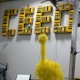# LabView examples: Programming structures

These examples (PDF) demonstrate the use of programming structures in LabVIEW.

Acceleration and Case Structure

• This example shows how to use shift registers and case structures to produce an effect similar to acceleration. If the sensor is pressed, the motors stop, but otherwise power is slowly added to the motors.

Basic Modulus

• This program shows the most basic use of the Quotient/Remainder block.  In other programming languages, this function is referred to as the Modulus, and allows the user to use the remainder of an equation.  In this example, the user inputs a number and the program determines (using the Modulus) if the number is even or odd.

Compute Factorial

• This program shows how to execute the concept of recursion (using a function within itself).  The factorial program takes in a number n, and computes n factorial (n!).  n! =  n*(n-1)*(n-2)*(n-3) etc.  By definition, 0! is equal to 1.   This example uses a while loop and a shift register.

Conditional Loop

• This example shows how to make a while loop dependent on a condition in order to keep running, instead of depending on a condition in order to stop.

Convert Polar to Cartesian

• This program takes a point in Polar coordinates and converts it into Cartesian Coordinates.

For Loops

• This example shows the use of a for loop, which is useful when you know precisely how many times a loop needs to be run.  The “N” in the top left corner represents the number of iterations the loop will go through.  In this particular example, the motor runs faster depending on which iteration the loop is on.

Shift Register Counting

• This example shows the basic use of a shift register in a while loop.  Shift registers are used to store values from the end of a loop and reuse them at the start of the loop.

Shift Registers and Timers

• This example demonstrates the use of both shift registers and timers.  After resetting the timer, the program reads how long the loop has been running, subtracts the last timer value, and displays the result to the screen, essentially measuring the run time of one iteration.

Using Sequences

• This example shows a flat sequence structure in action.

Waiting

• This example shows how to use the Wait For program.  The program first waits for a touch, moves forward, and then waits again for a touch to brake.

While Loop, Lamp Use

• This example shows the basic use of a while loop.  It also explains how to use the LED Lamps that are included in the Mindstorms kit.

## Resources

The following two tabs change content below.#### CEEO

The Tufts Center for Engineering Education and Outreach (CEEO) in Boston, Massachusetts, is dedicated to improving engineering education in the classroom, from Kindergarten to college. The Center houses faculty, staff, and graduate students from engineering disciplines and the education department.